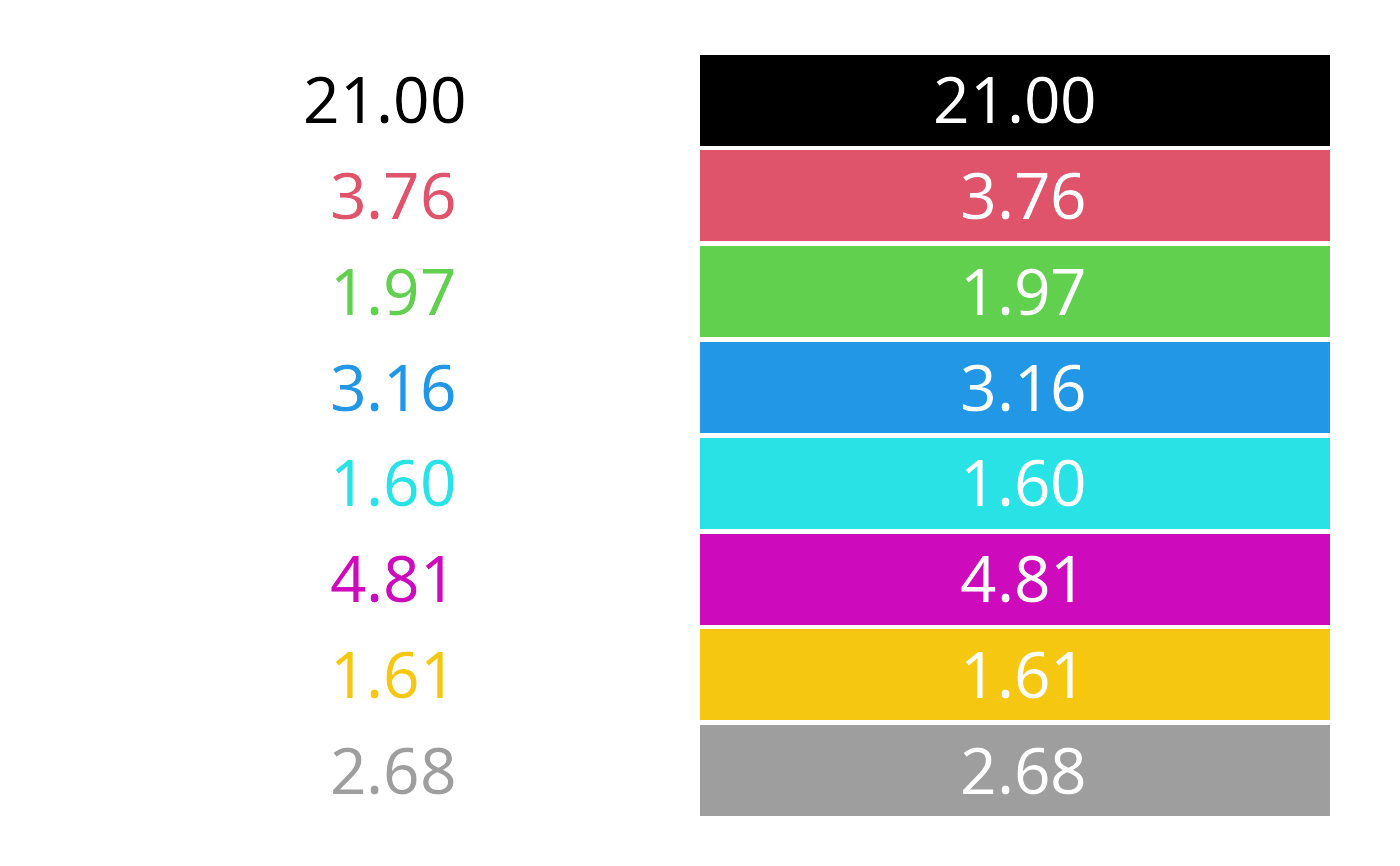Compute (and visualize) the contrast ratio of pairs of colors, as defined by the World Wide Web Consortium (W3C).

contrast_ratio(
col,
col2 = "white",
algorithm = c("WCAG", "APCA"),
plot = FALSE,
border = FALSE,
cex = 2,
off = 0.05,
mar = rep(0.5, 4),
digits = 2L,
...
)

## Arguments

col, col2

vectors of any of the three kind of R colors, i.e., either a color name (an element of colors), a hexadecimal string of the form "#rrggbb" or "#rrggbbaa" (see rgb), or an integer i meaning palette()[i]. Both can be vectors and are recycled as necessary.

algorithm

character specifying whether the established standard "WCAG" 2.1 algorithm should be used or the improved "APCA" 0.98G-4g algorithm, still under development.

plot

logical indicating whether the contrast ratios should also be visualized by simple color swatches. Can also be a vector of length 2, indicating whether the foreground color should be visualized on the background color and/or the background color on the foreground color.

border

logical or color specification for the borders around the color swatches (only used if plot = TRUE). The default is FALSE which is equivalent to "transparent". If TRUE the border is drawn in the same color as the text in the rectangle.

cex

numeric. Size of the text in the color color swatches (only if plot = TRUE).

off

numeric. Vertical offset between the different color swatches (only if plot = TRUE). Can also be of length 2 giving both vertical and horizontal offsets, respectively.

mar

numeric. Size of the margins around the color swatches (only if plot = TRUE).

digits

numeric. Number of digits for the contrast ratios displayed in the color swatches (only if plot = TRUE)

...

further arguments passed to the plot of the color swatches (only if plot = TRUE).

## Value

A numeric vector with the contrast ratios is returned (invisibly, if plot is TRUE).

## Details

The W3C Content Accessibility Guidelines (WCAG) recommend a contrast ratio of at least 4.5 for the color of regular text on the background color, and a ratio of at least 3 for large text. See https://www.w3.org/TR/WCAG21/#contrast-minimum.

The contrast ratio is defined in https://www.w3.org/TR/WCAG21/#dfn-contrast-ratio as (L1 + 0.05) / (L2 + 0.05) where L1 and L2 are the relative luminances (see https://www.w3.org/TR/WCAG21/#dfn-relative-luminance) of the lighter and darker colors, respectively. The relative luminances are weighted sums of scaled sRGB coordinates: 0.2126 * R + 0.7152 * G + 0.0722 * B where each of R, G, and B is defined as ifelse(RGB <= 0.03928, RGB/12.92, ((RGB + 0.055)/1.055)^2.4) based on the RGB coordinates between 0 and 1.

For use in the next major revision of the WCAG a new advanced perceptual contrast algorithm (APCA) has been proposed by Somers (2022), see also Muth (2022) for more background and details. APCA is still under development, here version 0.98G-4g is implemented. Unlike the standard WCAG algorithm, APCA takes into account which color is the text and which is the background. Hence for the APCA algorithm a matrix with normal and reverse polarity is returned. An absolute value of 45 is "sort of" like a WCAG ratio of 3, 60 is "sort of" like 4.5.

desaturate

## Examples

# check contrast ratio of default palette on white background
contrast_ratio(palette(), "white")
#>  21.000000  3.758588  1.973030  3.163940  1.603030  4.805641  1.608044
#>   2.679156

# visualize contrast ratio of default palette on white and black background
contrast_ratio(palette(), "white", plot = TRUE)contrast_ratio(palette()[-1], "black", plot = TRUE)# APCA algorithm
contrast_ratio(palette(), "white", algorithm = "APCA")
#>         normal    reverse
#> [1,] 106.04067 -107.88473
#> [2,]  64.27800  -69.77386
#> [3,]  37.72423  -42.10985
#> [4,]  58.24111  -63.64142
#> [5,]  26.62298  -30.08416
#> [6,]  71.04046  -76.51234
#> [7,]  27.09773  -30.60320
#> [8,]  52.07286  -57.27345
contrast_ratio(palette(), "white", algorithm = "APCA", plot = TRUE, digits = 0)